﻿ 基于因子分析和聚类分析研究云南省区域经济发展差异

# 基于因子分析和聚类分析研究云南省区域经济发展差异Research on the Regional Economic Development Differences in Yunnan Province Based on Factor Analysis and Cluster Analysis

Abstract: In order to scientifically evaluate the economic development level of various prefectures and cities in Yunnan Province, this paper selects 10 indicators from the statistical data of 16 cities in 2019 to analyze the economic development level of various regions in Yunnan Province. The factor analysis method is used to obtain the comprehensive score of each prefecture and city. From the score, there is a certain gap in the economic development level of the prefectures and cities in Yunnan Province. Kunming is the city with the best economic development level, and Nujiang Prefecture is the worst city. And then divide it into 6 categories by using hierarchical clustering method, and finally put forward corresponding suggestions for shortening this gap.

1. 引言

2. 文献综述

3. 相关理论

3.1. 因子分析

3.2. 聚类分析

4. 数据的分析

4.1. 数据的来源

4.2. 数据的描述性分析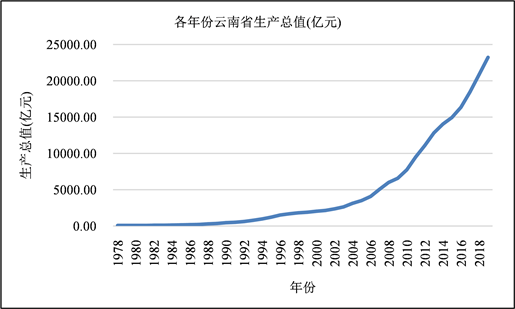Figure 1. Yunnan’s GDP in each year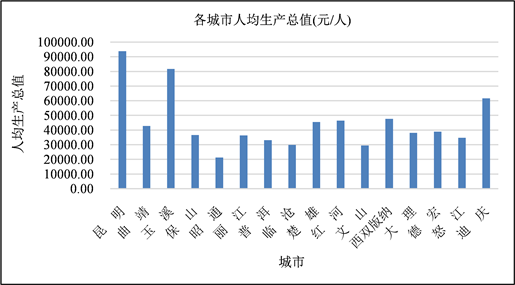Figure 2. Per capita GDP of each city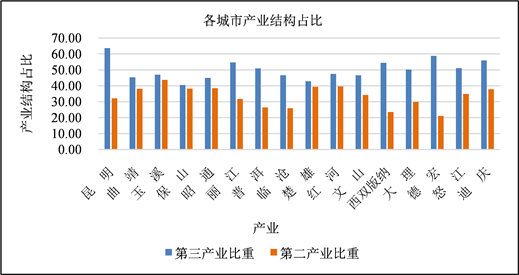Figure 3. Proportion of the industrial structure of each city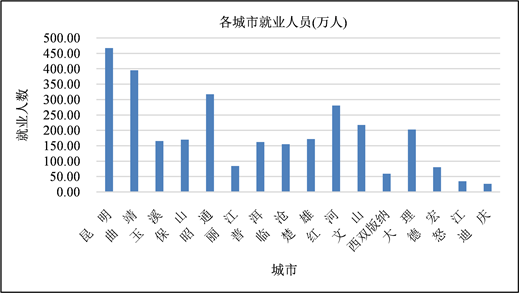Figure 4. Number of employees in each city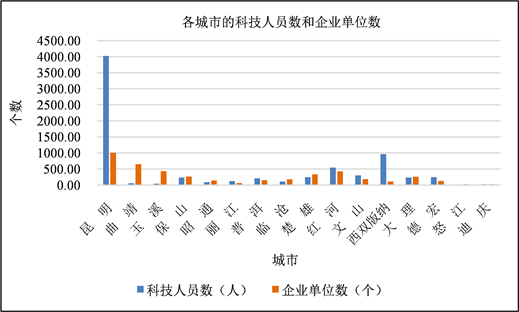Figure 5. Number of scientific and technological personnel and enterprise units in each city

4.3. 因子分析

4.3.1. 各指标间的相关矩阵Table 2. Correlation matrix between indicators

4.3.2. 因子分析的可行性检验

KMO检验统计量通常被用来衡量变量之间的相关系数和偏相关系数 。它的取值范围通常介于0和1之间。当KMO的值越趋近于1时，说明变量间的相关性就越强，因子分析对于这一数据而言就越适合；反之，当KMO的值越趋近于0时，说明变量间的相关性就越弱，因子分析对于这一数据而言就越不适合。

Bartlett的球形检验可以用来查验变量之间是否独立。如果变量之间独立(原假设)，则该数据不适合做因子分析，而如果变量之间不独立(备择假设)，则该数据适合做因子分析。Table 3. Results of two inspection methods

4.3.3. 基于主成分法的因子分析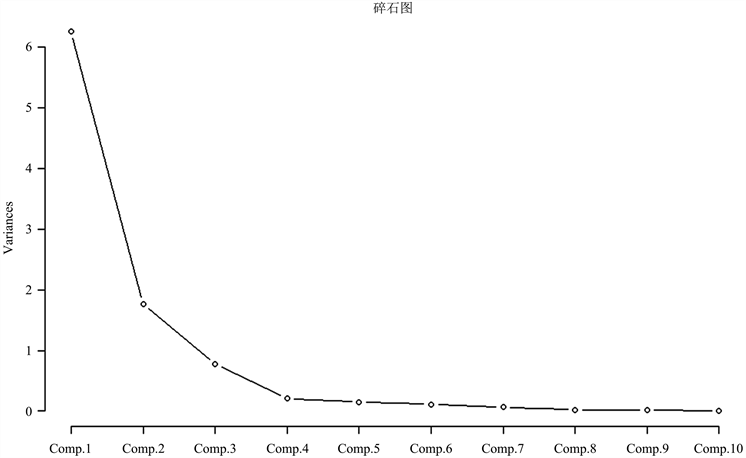Figure 6. Gravel mapTable 4. Variance contribution rate after rotation of each common factor$\begin{array}{l}{x}_{1}=0.902{f}_{1}+0.276{f}_{2}{}_{}{x}_{2}=0.992{f}_{1}+0.015{f}_{2}\\ {x}_{3}=0.992{f}_{1}-0.017{f}_{2}{}_{}{x}_{4}=0.901{f}_{1}+0.283{f}_{2}\\ {x}_{5}=0.431{f}_{1}+0.830{f}_{2}{}_{}{x}_{6}=0.127{f}_{1}-0.848{f}_{2}\\ {x}_{7}=0.750{f}_{1}+0.085{f}_{2}{}_{}{x}_{8}=0.760{f}_{1}-0.445{f}_{2}\\ {x}_{9}=0.914{f}_{1}+0.300{f}_{2}{}_{}{x}_{10}=0.926{f}_{1}-0.269{f}_{2}\end{array}$ (1)

$f=66.3%{f}_{1}+19.32%{f}_{2}$ (2)Table 6. Factor score and ranking

4.4. 聚类分析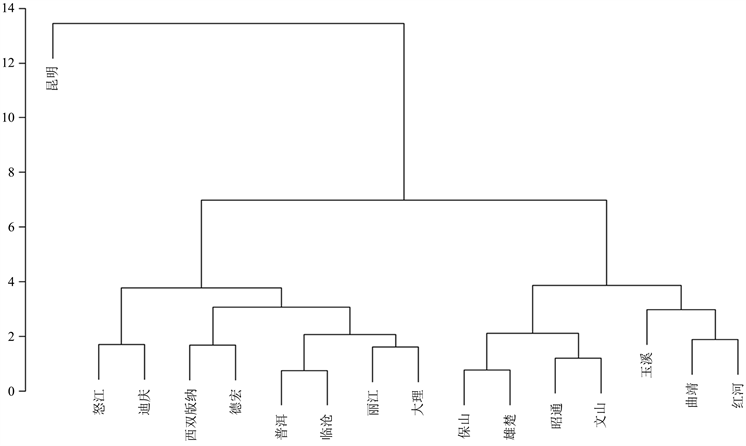Figure 7. Dendrogram of the economic development of 16 cities by the sum of squared deviation method and the clustering dendrogram

5. 结论

 吴波. 基于因子分析的珠三角地区经济差异实证研究[J]. 中国市场, 2013(20): 19-20.

 张自敏, 樊艳英, 陈冠萍, 等. 基于因子和聚类分析的广西区域经济发展差异研究[J]. 科技和产业, 2013, 13(2): 15-18, 43.

 石学刚, 汪戎, 吴春芳. 基于因子分析法的云南省各市(州)经济发展水平综合评价[J]. 现代商业, 2008(8): 226-227.

 费宇. 多元统计分析——基于R [M]. 北京: 中国人民大学出版社, 2014.

 黄春松. 长三角经济区、珠三角经济区与海西经济区的城市竞争力综合比较——基于因子分析模型的研究[J].经济社会体制比较, 2013(1): 205-216.

 张艳萍. 浅谈我国工业经济区域差异及协调发展[J]. 现代工业经济和信息化, 2014, 4(8): 11-12.

 薛薇. 基于SPSS的数据分析[M]. 北京: 人民大学出版社, 2006.

 吴海. 基于因子分析和熵权法的全国科技发展水平评价[J]. 经济师, 2016(1): 44-46.

 李翔. 加快云南连接东南亚, 南亚国际大通道公路建设步伐的探讨[J]. 云南现代交通, 2004, 1(1): 23-29.

 刘宪立, 杨蔚. 基于数据包络分析法云南低碳物流效率评价研究[J]. 现代商业, 2020(18): 124-125.

Top